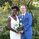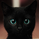จำนวนเข้าชม 460
The Center of Gravity Oscillator was created by John Ehlers (Cybernetic Analysis For Stocks And Futures pg 49) and this provides a pretty accurate way to see how the stock is trending. If the indicator stays above 0 then the stock is in a pretty strong uptrend and if it stays below 0 then the stock is in a pretty strong downtrend. Buy when the indicator changes from red to green and sell when it changes from green to red.

Let me know if you would like me to publish any other indicators or if you want something custom done!

## ความคิดเห็นhjsjshs
@hjsjshs, thank you! I took a long hiatus but I'm back for now. Hopefully the new pinescript changes will make my life easier now
ตอบกลับline #18, I had a different understanding here, I think it should be :" cg = denom != 0 ? (-num / denom) + (length / 2) : 0 " rather than "cg = denom != 0 ? (-num / denom) + ((length + 1) / 2) : 0", because index i start from zero, length is already the number of data + 1 already. What is your opinion?
ตอบกลับblackcat1402
@blackcat1402, This was actually how Ehlers wrote it and I believe he did it that way to give extra weight for the length in a way similar to how the ema is calculated. It has nothing to do with the index
ตอบกลับcheatcountry
@cheatcountry, i am confused because some prior mq4 codes use length, you are using length +1, and I had no idea on which is correct, FYI.

"
Num = 0.0;
Denom = 0.0;
for (int count=0; count 0) COG = Num/Denom - 0.5*(Length + 1);

Trigger = COG;
"

Here Length is equal to length-1, then (Length + 1) should be length too.

So, I am wondering several versions of prior arts using length, whether (length + 1) in your script should be changed into length. Hope I clearly illustrated the background of story :D
ตอบกลับblackcat1402
@blackcat1402, I think you are forgetting a few things. When do you loop of i = 0 to i = length - 1 and length is 10 then you are doing a total of 10 loops (0, 1, 2, 3, 4, 5, 6, 7, 8, 9) so in his formula he is getting the ratio of the total num by the total denom and then adding his custom constant at the end. Also there are many incorrect scripts out there because people aren't that great at programming and don't get it correct
ตอบกลับcheatcountry
@cheatcountry,

for(i=nLimit;i>=0 && !IsStopped();i--)
{
Num = 0.0;
Denom = 0.0;
for (int count=0; count<InpCGLength; count++)
{
Num += (1.0+count)*Price(i+count);
Denom += Price(i+count);
}
if (Denom != 0.0)
Cycle = -Num/Denom+(InpCGLength+1.0)/2.0;
else
Cycle = 0.0;